top of page

Forward Kinematics

Forward kinematics refers to the use of the kinematic equations of a robot to compute the position of the end-effector from specified values for the joint parameters.(Source Wiki)

Let me put in other way,

Given a kinematic chain composed of links and joints with multiple degree of freedom, finding the position and orientation of the end-effector in the operational workspace when all the joint parameters are known. This is called FORWARD KINEMATICS.

If you did not understood the above definitions or what is kinematic chain, DOF or endeffector, don't worry throughout this blog I will clear all your doubts. If you have understood everything at this point then you are ready to go for inverse kinematics.

See the below fig 1, it will give you an intuition on what is joint, link and kinematic chain.Fig. 1 Example to illustrate Joints, Links, End-effector, Kinematic chains

Joints: Joints are the movable parts(actuators) of the robots that connects the links of the robot. Joints cause relative motion between adjacent links.

Kinematic chains:  Kinematic chain is the assembly of links connected by joints to produce a desired motion. Human arm is an example of a kinematic chain. Human body is a group of kinematic chains connected in series.

Degree of Freedom (DOF): Degree of freedom in robotic is simply the total number of independent joints which can change the  pose of the robot. If you take a human arm from shoulder to palm (fingers not included), arm has 7 DOF. Try to identify the joints yourself (Hint: Shoulder alone has 3 DOF).

End-Effector: End-effector is the last link of the robot arm or manipulator that interacts with the environment. The last link can be gripper tool to do pick and place actions or a welding gun or simply a magnet.

Workspace:  Workspace is the space in which your robot can work i.e., all reachable points by the robot's endeffector constitutes to workspace.

Below is a example of two link planar arms. The blue area in the fig 2 is the workspace of the arm.Fig. 2 Workspace of two different 2-link planar manipulators

Joint parameters: Joint parameters is nothing but the state value of the joint. This state value depends on the type of the Joint.

Type of Joints:

Most commonly used joints in robotics are revolute joint, prismatic joint and continuous joint.

• Revolute - a hinge joint that rotates along the axis and has a limited range specified by the upper and lower limits.

Ex: DC servo motor which can rotate form 0 - 180 or 0 - 360

• Prismatic - a sliding joint that slides along the axis, and has a limited range specified by the upper and lower limits.

Ex: slider joint, conveyor belt.

Units: meters

• Continuous - a continuous hinge joint that rotates around the axis and has no upper and lower limits.

Ex: DC motor which can rotate continuously in clockwise or anti-clockwise direction.

At this point you should be able to understand the definition of Forward Kinematics.

Now lets find out how to calculate FK for below robot.Fig. 3 RRR Manipulator

This robot is known as RRR manipulator since it consists of 3 revolute joints. The black dotted lines represent the axis of rotation of the joints. This is the home position of the manipulator where all the actuators(Joints) are at zero positions.

The first step in deriving forward kinematics is to assign frames to the links.

If you have done any course on robotics or you have studied any books on robotics then you might definitely heard about DH parameters. DH parameter are 4 parameters used to assign frames to links of a robot. DH convention will describe the rotation and translation of each link in terms of 4 parameters (namely, the link length, twist, offset, and the joint angle) instead of 6 (3 for translation, 3 for rotation). Earlier computers at that time were not so advanced and fast as today so the calculations to be as simple as possible so almost all robots used DH parameters to attach links and find the forward kinematics. But to use DH parameters you have to follow some rules in attaching frames to the links.

Now-a-day's DH-convention has become a legacy concept. Today computers are most advanced and fast, 6 parameters for each link is not a big deal. Now we are free to attach the frames to the link as you wish. So I am not going to follow DH convention rather I will attach all the frames in such a way that orientation of all the frames are same when joint angle of the joints are  zero.

I will attach the frame to the links as shown fig 4.Fig. 4 Frames assigned to RRR Manipulator

As you can see I have attached a frame {0} to the base because I want to know the position of the end-effector from that point. Frame {0} is the reference frame and it is fixed frame. Also I have attached a frame {ee} at the tip of the 3rd link which represents the end-effector frame. I have attached frames {1},{2},{3} to link1, link2 and link3 respectively.

NOTE: By seeing figure you may be thinking that I am attaching frames to joints instead of links. Don't get confused the frames are attached to links but it is recommended to attach frames to the links at the axis of the joint.

OK!!! we are done with frame assignment. Then the next step is calculate the transformation of the adjacent frames i.e., T01, T12, T23, T3-ee.

Let say θ1, θ2, θ3  are the joint parameters for joint1, joint2, joint3, respectively.

T01: This is the transformation of link1 frame {1} w.r.t Base frame {0}.  As you can see from the diagram, first, frame {1} is b units far from {0}  in z-direction and 0 units in x, y directions i.e., first  {1} translates w.r.t {0}. Next link1 can rotates about joint1 in z direction, there exists a change in orientation along z-direction.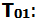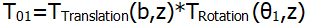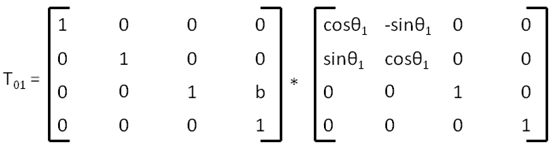From now on I will write cosθ and sinθ in short forms like below

cosθ1: c1, sinθ1: s1 ; cosθ2: c2, sinθ2: s2  ; cosθ3: c3, sinθ3: s3 ; cosθ: c, sinθ: s

cosθ1* sinθ1 =c1s1 ; cosθ1* sinθ2 =c1s2 ; cosθ2* sinθ1 =c2s1 ; so on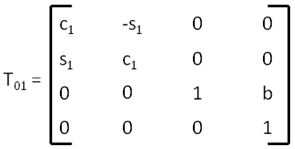T12: This is the transformation of link2 frame {2} w.r.t link1 frame {1}.  Frame {2} first translate in z-direction about L1 units and then rotates about joint 2 in x-directions w.r.t frame {1}.T23: This is the transformation of link3 frame {3} w.r.t link2 frame {2}.  Frame {3} first translate in y-direction about L2 units and then rotates about joint 3 in x-directions w.r.t frame {2}.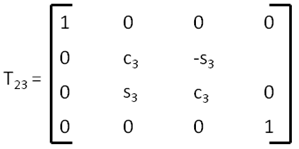T3-ee: This is the transformation of end-effector frame {ee} w.r.t link3 frame {3}.  Frame {ee} only translate along y-direction about L3 units.Ok we have found all the adjacent frames transformation matrices. Now we have to find the transformation of end-effector frame {ee} w.r.t base frame {0} by multiplying T01, T12, T23, T3-ee in order.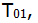After doing boring matrix multiplication we get,This 4X4 transformation matrix T0ee is the forward kinematics of our RRR manipulator.

We can find the position [x,y,z] and orientation [R] of the endeffector w.r.t base by substituting joint parameters θ1, θ2 and θ3.Equations for PositonPosition:Orientation: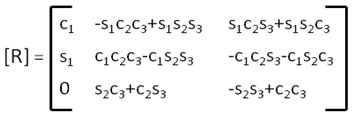As said earlier, in home pose θ1, θ2 and θ3.   are 0,0,0. Substitute this values and find endeffector position and orientation in home pose.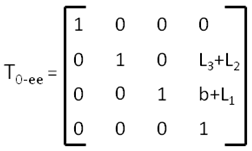Lets verify the resultant transform matrix with the physical robot as shown in fig 5.

As you can see, the rotation part in Transform matrix is identity, that means the orientation of end-effector is same as base orientation. This is true because we have attached the frames such that at home pose all the frames are in same orientation.Fig. 5 RRR Manipulator's pose in Home position

Coming to the translation part, our transformation matrix says end-effector is 0 units far in x-direction, L2+L3 in y-direction and b+L1 in z-direction from base. This is also true we can verify from fig 5.

In this way we test the transformation matrix by putting the manipulator in some known configurations (like θ1=0, θ2=90, θ3=0  ==> x=0,y=0,z=b+L1+L2+L3        ) and make sure that we haven't commit any mistake in matrix multiplication or frame assignments.

Note: Rotation matrix can also be known intuitively. To know how, check out this link

For                                 , the transformation matrix looks like below and robot looks as shown in fig 6.Fig. 6 RRR Manipulator's pose whenSome random poses of the manipulator are shown in fig 7 and fig 8. For these poses we cannot say intuitively the position and orientation of the end-effector just by looking it. We need to use transform matrix/ Forward Kinematics,          to find the pose of the endeffector. Try to solve by your self.Fig. 7 RRR Manipulator's pose whenFig. 8 RRR Manipulator's pose whenHurrah....!!!!! Now you know how to find the forward kinematics of a robot.

But don't be happy so early, try to solve FK for a 6-DOF robot shown in fig 9,Fig. 9 6-DOF Manipulator

Let me summarise the steps to find forward kinematics of a robot.

1. First you have to attach frames to the links when the robot is in home position.

2. Then calculate the transformation matrices of the adjacent frames from base to the end-effector.

3. Next, multiply all the transformation matrices in correct order to get the final transform matrix T0ee

4. Substitute the given joint values in the T0ee matrix to get the end-effector position and orientation with respect to the base/reference frame.

If you got stuck at any point in computing FK for this 6-DOF bot then feel free to comment your question in the comment section below or send your question through "Contact Us", we will reply you as soon as possible.

If you have found it correctly, Congratulations.!!!! you have understood how to attach frames and compute FK.

Thank you. See you in Inverse Kinematics.bottom of page